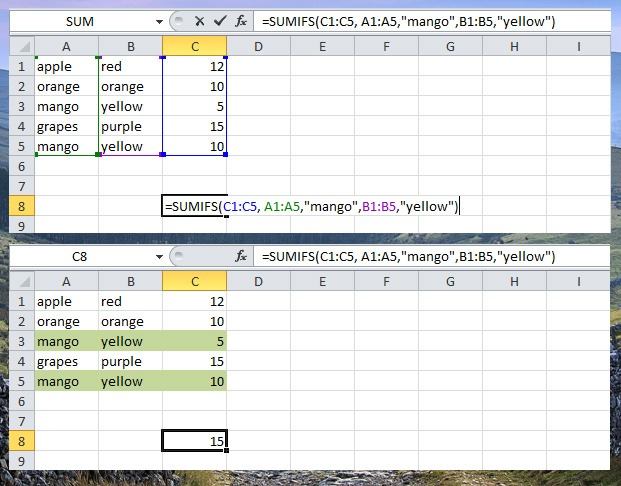# Count and Sum Functions

Count and Sum are one of the most used functions in Excel. You can easily count or sum the content of the worksheet based on one or multiple criteria.

There are 6 functions included in count and sum (Count, Countif, Countifs, Sum, Sumif and Sumifs).

Count

Use this function to count the number of cells that contain numbers. Here’s how to use COUNT function.

Position the active cell where you want the output to appear, then type this =COUNT(use your mouse to select the cells to countas you can see in the left picture in the example screenshot below. Then press enter to automatically count.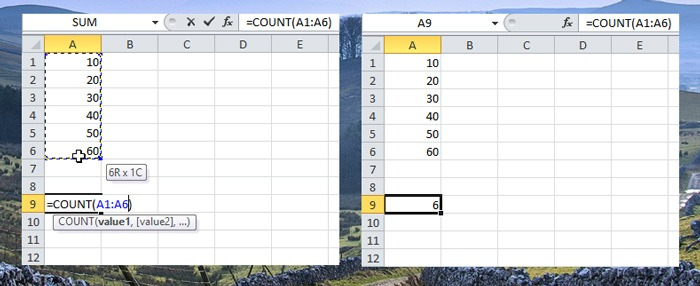Countif

Countif use to count the number of cells with number based on one criteria. In the sample screenshot below, I count the number of cells with higher than 35 value.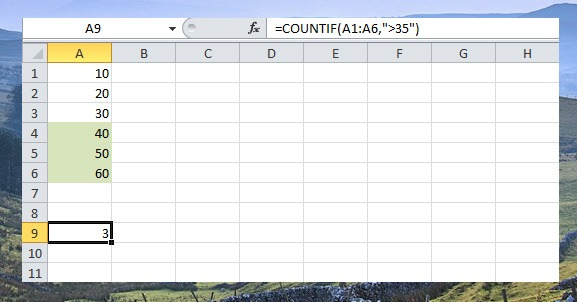Countifs

Countifs use to count cells with number based on multiple criteria. In the example I will count the mango with more than 4 value.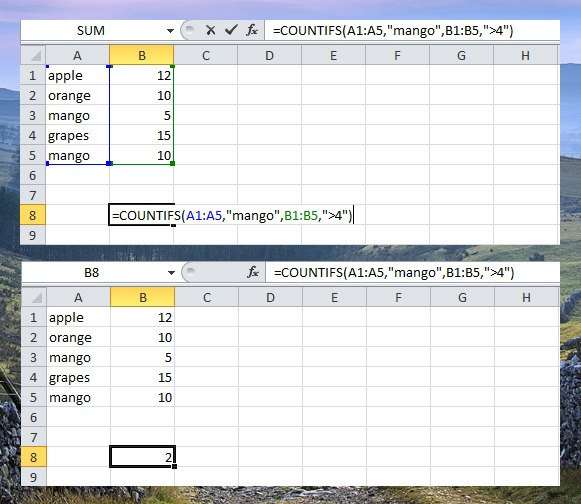Sum

Adding the value of a range of cells is easy using SUM functions. Simply position the active cell where you want to put the sum, then type =SUM(use your mouse to select the cells to add) then press enter.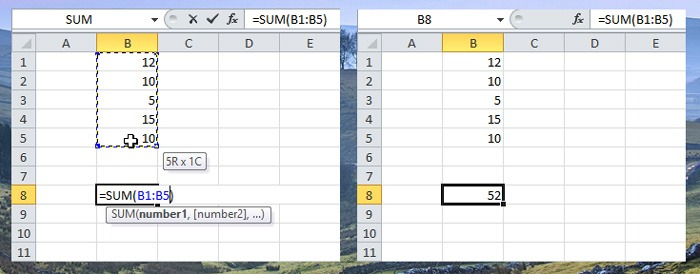Sumif

Add the cell’s value based on one criteria. Example, add the cell with value more than 10.

To add the cells based on one criteria, example “mango” use this SUMIF function, (three arguments, first is the range where criteria is based, second is the criteria then last argument is the range to sum).Sumifs

To add the cells based on multiple criteria, for example, mango and yellow), use the following SUMIFS function (first argument is the range to sum).